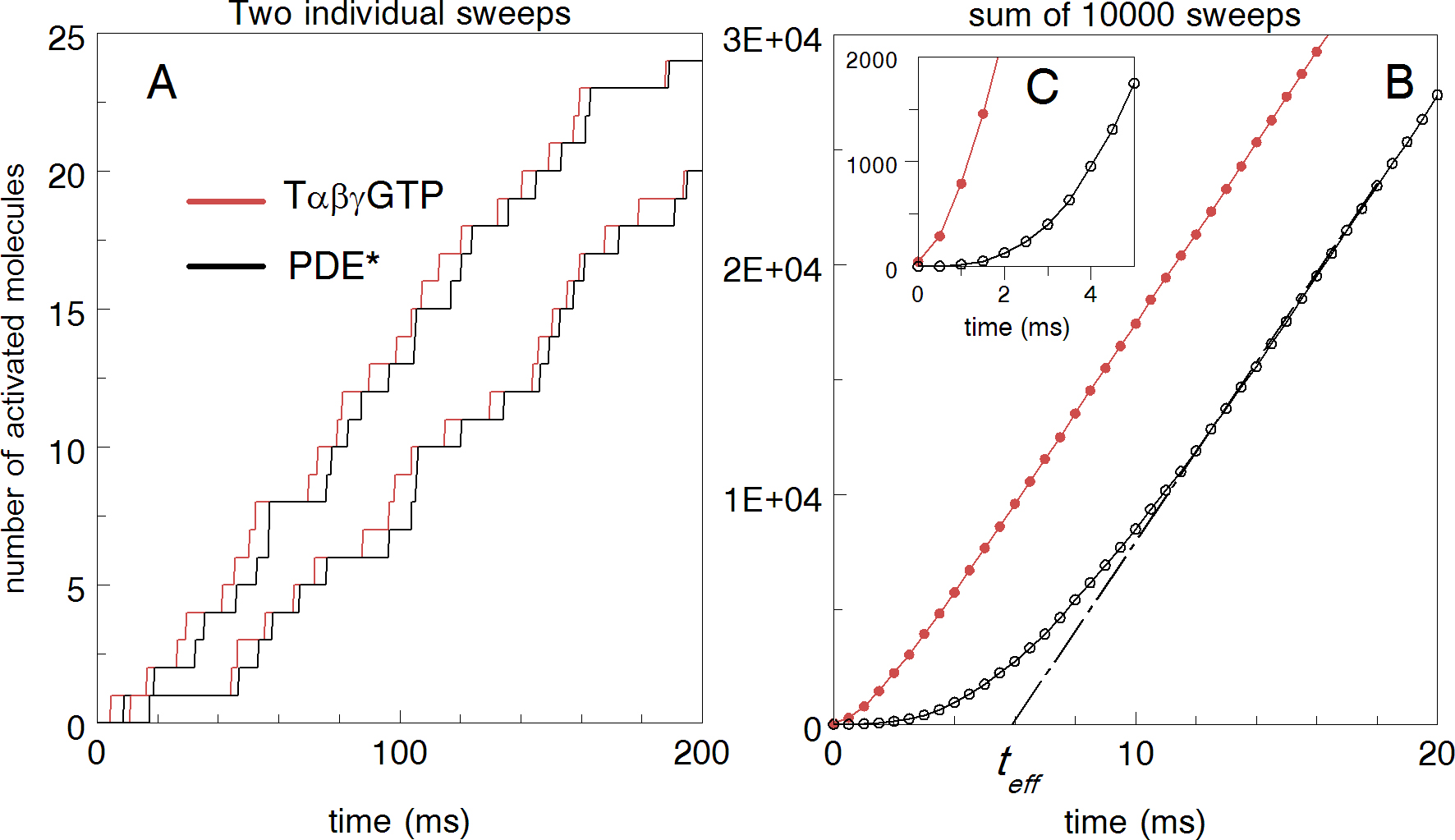Figure 4 of Rotov, Mol Vis 2017; 23:416-430.

Figure 4. Time courses of active transducin (T*) and phosphodiesterase (PDE*) determined with a stochastic simulation. A: Two independent repetitive cycles of reactions show the time course of accumulated concentrations of Tαβγ-GTP and PDE* after the activation of single rhodopsin. B: The whole cycle is repeated 10,000 times to get the result summed over many quanta. Time constants (in ms) are t0 = 1, t1 = 4.7, t2 = 0.1, t3 = 0.1, t4 = 0.1. Total duration of the activation cycle (t1 + t2 + t3 + t4 = 5 ms) was fixed according to biochemical data on the rate of T activation in frog rods (about 200 s−1 ). t5 = 2.5; t6 = 2.5. t0 is the time of Meta II production in frog rods .